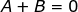## Monday, April 13, 2015

### Separation of Variables, 41

Category: Differential Equations

"Published in Newark, California, USA"

Find the general solution for

Solution:

Consider the given equation above

Express y' as dy/dx as follows

By separation of variables, transpose dx and (e2x + 4) to the right side of the equation and y to the left side of the equation, we have

Integrate on both sides of the equation, we have

Did you notice that the right side of an equation cannot be integrated by simple integration? The differential of e2x + 4 is 2e2xdx and 2e2x is missing at the numerator. Because of this, we have to use the algebraic substitution as follows

LetSo thatThe value of dx isHence, the above equation becomes

By separating the partial fractions, we can solve for the values of A and B as follows

Equate x0:Equate x:Substitute the values of A and B to the above equation, we have

Take the inverse natural logarithm on both sides of the equation, we have

butHence, the above equation becomes

Therefore, the general solution is